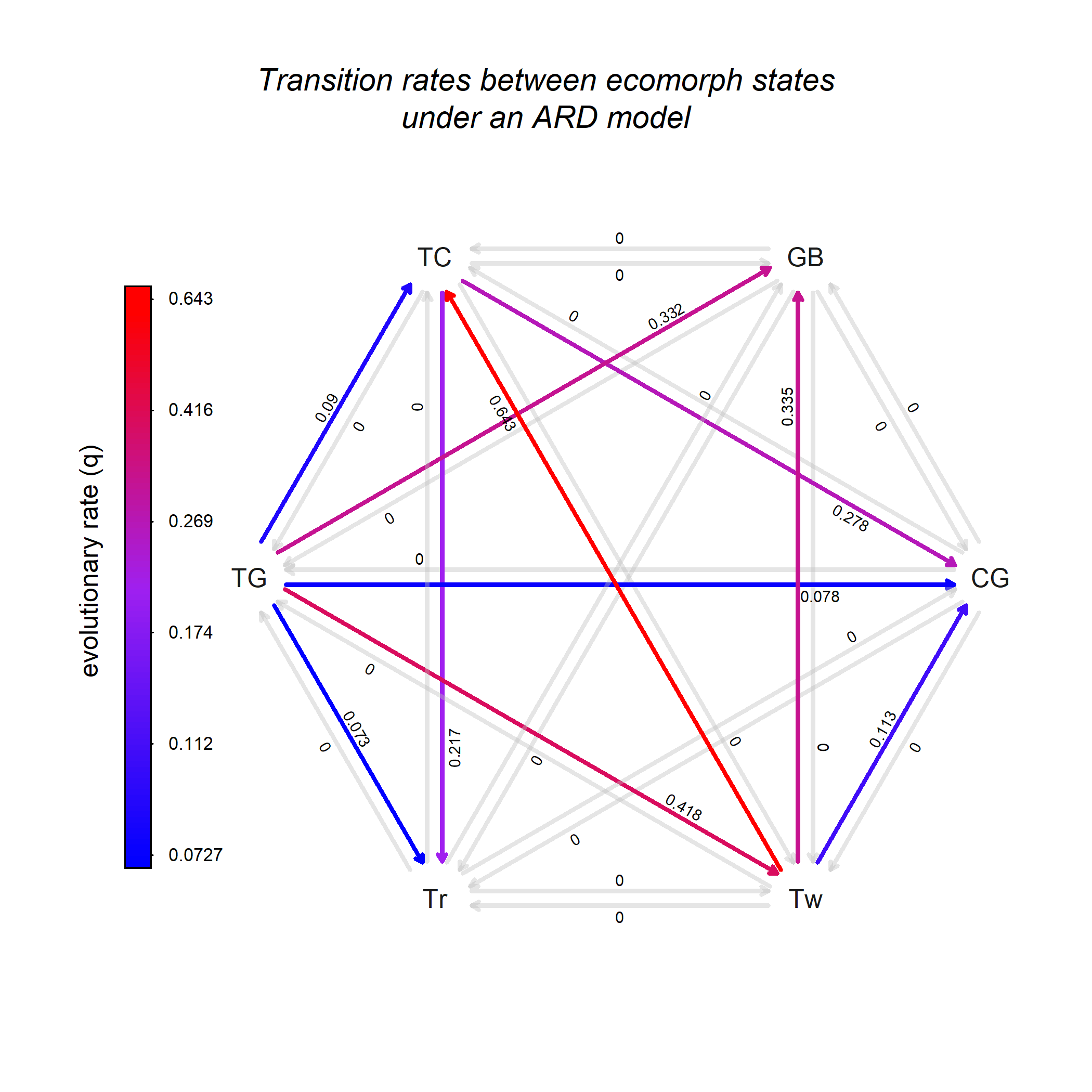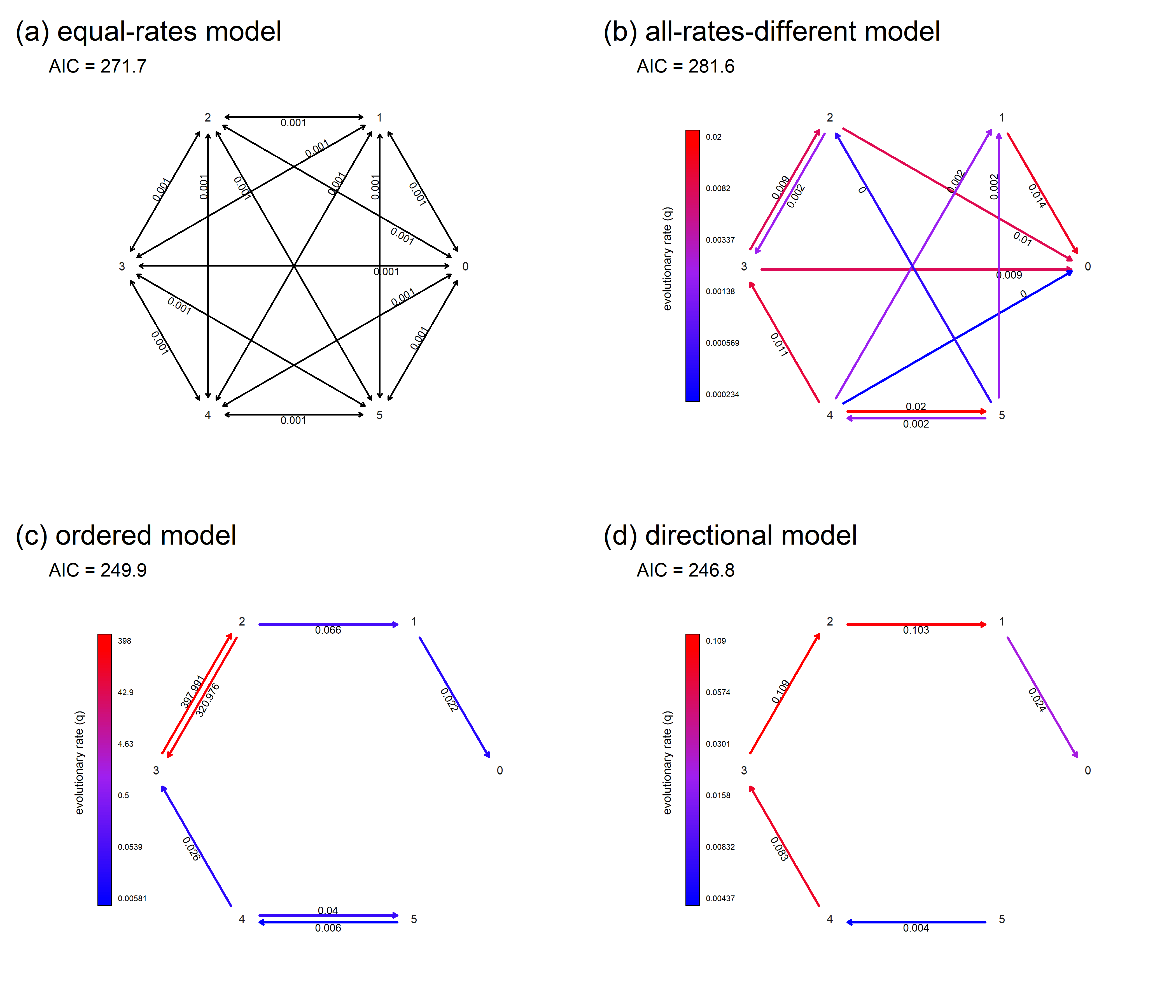## Tuesday, August 30, 2022

### Plotting a fitted Mk model coloring the transition types by rate

This morning I was scrolling through old posts to look for a new background image for my blog, when I stumbled on this post showing how to plot a fitted Q matrix with arrows colored by rate.

What?

I totally forgot that phytools could do that!

In fact, in preparing a recent talk for ESEB, I'd been thinking how great it would be if exactly such a method existed.

Since I'd forgotten, and since we don't show it in our upcoming book, I thought it was probably worth revisiting here.

Here's the example from the original post showing transitions between discrete 'ecomorph' conditions in Anolis lizards.

``````## load phytools
library(phytools)
row.names=1)
ecomorph.tree<-drop.tip(anole.tree,
geiger::name.check(anole.tree,anole.ecomorphs)\$tree_not_data)
ecomorph<-setNames(anole.ecomorphs\$ecomorph,row.names(anole.ecomorphs))
## fit ARD model
fitARD<-fitMk(ecomorph.tree,ecomorph,model="ARD",pi="fitzjohn")
fitARD
``````
``````## Object of class "fitMk".
##
## Fitted (or set) value of Q:
##          CG       GB        TC        TG       Tr        Tw
## CG 0.000000 0.000000  0.000000  0.000000 0.000000  0.000000
## GB 0.000000 0.000000  0.000000  0.000000 0.000000  0.000000
## TC 0.278023 0.000000 -0.495184  0.000000 0.217161  0.000000
## TG 0.078225 0.332274  0.090038 -0.991693 0.072734  0.418422
## Tr 0.000000 0.000000  0.000000  0.000000 0.000000  0.000000
## Tw 0.112733 0.334595  0.642925  0.000000 0.000000 -1.090252
##
## Fitted (or set) value of pi:
## CG GB TC TG Tr Tw
##  0  0  0  1  0  0
## due to treating the root prior as (a) nuisance.
##
## Log-likelihood: -68.226274
##
## Optimization method used was "nlminb"
``````
``````## create plot
plot(fitARD,color=TRUE,lwd=3)
title(main="Transition rates between ecomorph states\nunder an ARD model",
font.main=3,line=-1)
``````Holy cow! Isn't that so cool?

For fun, let's try the digit number evolution example from our book using data taken from Brandley et al. (2008).

In this example, we fit four different models: an equal-rates (“ER”) model, an all-rates-different (“ARD”) model, and two different ordered evolution models.

``````## read data matrix
## name.check
chk<-geiger::name.check(sqTree,sqData)
## drop tips of tree that are missing from data matrix
sqTree.pruned<-drop.tip(sqTree,chk\$tree_not_data)
## drop rows of matrix that are missing from tree
sqData.pruned<-sqData[!(rownames(sqData)%in%
chk\$data_not_tree),,drop=FALSE]
## extract trait
toes<-setNames(as.factor(sqData.pruned[,"rear.toes"]),
rownames(sqData.pruned))
## fit models
fitER<-fitMk(sqTree.pruned,toes,model="ER",pi="fitzjohn")
fitARD<-fitMk(sqTree.pruned,toes,model="ARD",pi="fitzjohn")
ordered.model<-matrix(c(
0,1,0,0,0,0,
2,0,3,0,0,0,
0,4,0,5,0,0,
0,0,6,0,7,0,
0,0,0,8,0,9,
0,0,0,0,10,0),6,6,byrow=TRUE,
dimnames=list(0:5,0:5))
directional.model<-matrix(c(
0,0,0,0,0,0,
1,0,0,0,0,0,
0,2,0,0,0,0,
0,0,3,0,0,0,
0,0,0,4,0,0,
0,0,0,0,5,0),6,6,byrow=TRUE,
dimnames=list(0:5,0:5))
fitOrdered<-fitMk(sqTree.pruned,toes,model=ordered.model,
pi="fitzjohn")
fitDirectional<-fitMk(sqTree.pruned,toes,model=directional.model,
pi="fitzjohn")
AIC(fitER,fitARD,fitOrdered,fitDirectional)
``````
``````##                df      AIC
## fitER           1 271.6501
## fitARD         30 281.6477
## fitOrdered     10 249.9346
## fitDirectional  5 246.7741
``````

Now let's plot them all. For the `"ER"` model we aren't able to use `color=TRUE` since all transitions in Q have the same rate. We'll use it for all the other models, and also set `show.zeros=TRUE`.

``````## create plot
par(mfrow=c(2,2))
plot(fitER,cex.rates=0.9,lwd=2)
legend("topleft",legend=paste("AIC =",round(AIC(fitER),1)),bty="n",
cex=1.6)
plot(fitARD,cex.rates=0.9,show.zeros=FALSE,lwd=3,color=TRUE)
legend("topleft",legend=paste("AIC =",round(AIC(fitARD),1)),bty="n",
cex=1.6)
plot(fitOrdered,cex.rates=0.9,show.zeros=FALSE,lwd=3,color=TRUE)
legend("topleft",legend=paste("AIC =",round(AIC(fitOrdered),1)),bty="n",
cex=1.6)
plot(fitDirectional,cex.rates=0.9,show.zeros=FALSE,lwd=3,color=TRUE)
legend("topleft",legend=paste("AIC =",round(AIC(fitDirectional),1)),bty="n",
cex=1.6)